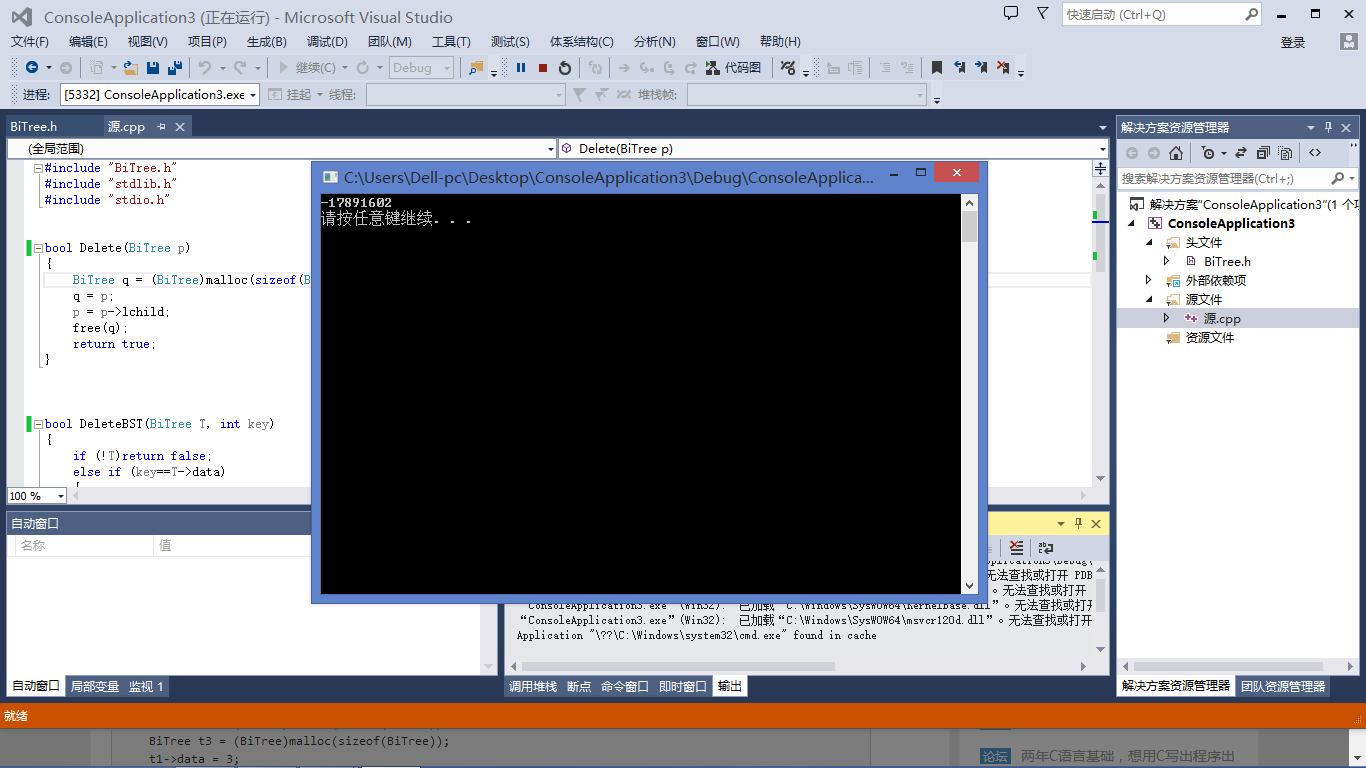c语言，形参是指针的引用，和形参是指针，两者结果不同，求指点

`````` typedef struct BiTNode
{
int data;
struct BiTNode *lchild, *rchild;

}BiTNode, *BiTree;
``````

`````` #include "BiTree.h"
#include "stdlib.h"
#include "stdio.h"

bool Delete(BiTree &p)
{
BiTree q = (BiTree)malloc(sizeof(BiTNode));
q = p;
p = p->lchild;
free(q);
return true;
}

bool DeleteBST(BiTree &T, int key)
{
if (!T)return false;
else if (key==T->data)
{
return Delete(T);
}
else if (key < T->data)
{
return DeleteBST(T->lchild, key);
}
}

int main()
{
BiTree t1 = (BiTree)malloc(sizeof(BiTNode));
BiTree t2 = (BiTree)malloc(sizeof(BiTNode));
BiTree t3 = (BiTree)malloc(sizeof(BiTNode));
t1->data = 3;
t2->data = 2;
t3->data = 1;
t1->lchild = t2;
t1->rchild = NULL;
t2->lchild = t3;
t2->rchild = NULL;
DeleteBST(t1, 2);

printf("%d\n", t1->lchild->data);

system("PAUSE");

return 0;

}

``````

`````` #include "BiTree.h"
#include "stdlib.h"
#include "stdio.h"

bool Delete(BiTree p)
{
BiTree q = (BiTree)malloc(sizeof(BiTNode));
q = p;
p = p->lchild;
free(q);
return true;
}

bool DeleteBST(BiTree T, int key)
{
if (!T)return false;
else if (key==T->data)
{
return Delete(T);
}
else if (key < T->data)
{
return DeleteBST(T->lchild, key);
}
}

int main()
{
BiTree t1 = (BiTree)malloc(sizeof(BiTNode));
BiTree t2 = (BiTree)malloc(sizeof(BiTNode));
BiTree t3 = (BiTree)malloc(sizeof(BiTNode));
t1->data = 3;
t2->data = 2;
t3->data = 1;
t1->lchild = t2;
t1->rchild = NULL;
t2->lchild = t3;
t2->rchild = NULL;
DeleteBST(t1, 2);

printf("%d\n", t1->lchild->data);

system("PAUSE");

return 0;

}

``````1个回答C语言中的形参是指针的传参技巧

C语言中指针作为形参传递数据的问题

#include "stdafx.h" void cmdsend(unsigned int * info); int main() { unsigned int senddata; senddata = 8; senddata = 2; senddata = 0; senddata = 4; senddata = 5; cmdsend( senddata ); while(1){;} } void cmdsend(unsigned int * info) { unsigned int k; for (k = 0; ((*info) != '\0') && (info != NULL); *info++, k++) printf("senddata is %d\r\n", *info); } 如上面程序所示，我想将数组sendata完整的输出，现在只能打印出8，2.即在sendata处结束，好像判断为 info == NULL了，请问应该怎样修改，才能输出完整数组？在使用 *info 指针作为形参的前提下。

#include<stdio.h> int s(int *a,int *b) { int t; t=*a; *a=*b; *b=t; } main() { int a,b; int *p1,*p2; scanf("%d,%d",&a,&b); p1=&a; p2=&b; if (a<b) s(p1,p2); printf("%d,%d",*p1,*p2); } 这是代码1。 ``` swap(int *p1,int *p2) {int *p; p=p1; p1=p2; p2=p; } main() { int a,b; int *pointer_1,*pointer_2; scanf("%d,%d",&a,&b); pointer_1=&a;pointer_2=&b; if(a<b) swap(pointer_1,pointer_2); printf("\n%d,%d\n",*pointer_1,*pointer_2); } 这是代码2。 ``` #include<stdio.h> int s(int a,int b) { int temp; temp=a; a=b; b=temp; } main() { int a,b; a=3;b=4; s(a,b); printf("%d,%d",a,b); } 这是代码3。 下面的是我对这三个代码的理解 代码1和代码2的区别是，代码1指针地址不变，而指针地址里的内容发生了改变。代码2的地址里的内容不发生改变而是两个指针交换地址。 代码3没有指针只是一般的交换。 然后我就不理解了，如果说是形参在函数调用完毕就释放而且没有return语句的话，主函数的值应当不发生改变。这样的话，代码1的主函数的实参数值交换没法解释。当然代码2和3按照这个逻辑是可以解释的。还有代码1的swap函数里的间接调用 和代码3的直接调用这样有区别吗？

C语言函数的形参（结构体类型）和实参类型（指针）可以不同吗？

int numstus; int lens; student *pointer; void main() { int i=1; char str; lens=100; pointer=(student *)malloc(lens*LEN); /*分配内存*/ numstus=0; welcome(); /*欢迎界面*/ while(i>0) { i=menu_select(); /*控制菜单*/ switch(i) { case 1:addrecord(pointer);break; /*增加学生信息*/ 。。。省略 而当调用addrecord函数的定义是 void addrecord(student stud[]) {...} pointer是个结构体指针 addrecord参数是 结构体类型 程序无错，传递可行 真是怎么回事

/*3. 输入两个整数，设计两个功能，输入1调用max函数，求出这两个数字中的大的那一个； 输入2调用min函数，求出这两个数字中的小的那一个。要求用到函数指针。*/ ``` #include <iostream> using namespace std; /* run this program using the console pauser or add your own getch, system("pause") or input loop */ int max(int x,int y) { return x>=y?x:y; } int min(int x,int y) { return x<=y?x:y; } int main(int argc, char** argv) { int x,y; cout<<"请输入两个整数"<<endl; cin>>x>>y; cout<<"请输入调用函数"<<endl; int i=0; cin>>i; int (*functionPointer)(int,int); //定义函数指针 if(i==1) { functionPointer=max; } else functionPointer=min; cout<<functionPointer(x,y); return 0; } ```

C语言函数传参：如何实现指针参数引用传递

C语言中指针数组作为函数参数传递的地址问题？

c语言中函数形参为空指针，调用时可以不用带实参吗？

c语言二维指针作为参数的问题。

#include<stdio.h> int stringcut(char *str, char dest[],int num,int lines) /*如果这里不加上50会出错*/ { char *temp=str; int k=0; if(strlen(str) ==NULL) { return -1; } for(k=0;k<lines;k++) { strncpy(dest[k],temp,num); temp=temp+num; } return 0; } main() { char str[]="abcdefghijklmnopqrstuvwxyz"; char dest; int ret; memset(dest,0,150); ret=stringcut(str,dest,5,3); if(ret!=0) { printf("failse\n"); } printf("[%s]\n",dest); printf("[%s]\n",dest); printf("[%s]\n",dest); } 请问写一个函数为二维数组赋值的时候，二维数组作为参数，应该怎么写，为什么？上面注释的地方为什么去掉50的时候就报错？ 不知道为什么参数不能写成char **dest,如果这样，程序会崩溃！

C语言函数不能返回局部变量或者形参的地址？为什么

#include <stdio.h> int *func(int x,int y,int z) /*返回地址值的函数*/ { int max; if(x>=y&&x>=z) return(&x); if(y>=x&&y>=z) return(&y); return(&z); } main() { int a,b,c,*p; printf("\nPlease input a,b,c:\n"); scanf("%d%d%d\n",&a,&b,&c); p=func(a,b,c); printf("a=%d,b=%d,c=%d,*p=%d\n",a,b,c,*p); } 例如这题， ``` ```

C语言数组作为参数会退化为指针，这是什么意思？

C语言数组作为参数会退化为指针，这是什么意思？指针和数组的不同是什么？

c语言(void *)利用指针传递参数出错

labwindowscvi编写的函数，void function(void * a)；利用指针a传递参数失败，在单步执行时发现，进入该函数后，a指针所指向的内容乱掉，不是调用前给a指针所赋的值，求大神指点，别说放弃用指针之类的建议！

【C语言】有关数组转置与指针应用的题目，求大佬解答

C语言指针数组问题，为什么可以不指定长度

int (*a)表示一个指向一个整形数组的指针这个好理解，但是为什么int (*a)[]可以编译通过呢

c语言中数组的指针表示法

MySQL数据库面试题（2020最新版）

HashMap底层实现原理，红黑树，B+树，B树的结构原理 Spring的AOP和IOC是什么？它们常见的使用场景有哪些？Spring事务，事务的属性，传播行为，数据库隔离级别 Spring和SpringMVC，MyBatis以及SpringBoot的注解分别有哪些？SpringMVC的工作原理，SpringBoot框架的优点，MyBatis框架的优点 SpringCould组件有哪些，他们...

Python爬虫，高清美图我全都要（彼岸桌面壁纸）

6年开发经验女程序员，面试京东Java岗要求薪资28K

Java岗开发3年，公司临时抽查算法，离职后这几题我记一辈子